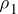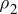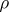# Day 91

When a fluid is in motion, its flow can be characterized one of two ways:

1. Streamline or laminar; every particle that passes a particular point moves exactly along the smooth path followed by particles that passed the point earlier.
2. Turbulent irregular flow; irregular motions (eddy currents) may be present; observed when there are abrupt changes in the velocity of the fluid.

(Viscosity here refers to the degree of internal friction in the fluid.)

For an ideal fluid:

1. The fluid is nonviscuous (no internal friction force between layers).
2. The fluid is incompressible (density is constant).
3. The fluid motion is steady (velocity, density, and pressure at each point do
not change.
4. The fluid moves without turbulence (each element of the fluid has zero angular velocity about its center; no eddy currents).

Changes in the velocity of the fluid and/or the size and shape of the pipe through which it is flowing can disrupt the flow. The product of the cross-sectional area of the pipe and the fluid speed at that cross section is a constant. This means that where the pipe is constricted, the velocity is high and vice versa.

This is known as the equation of continuity.A1v1 =A2v2

(= density, A = area, v = velocity)

The product of A X v is called the flow rate. The amount of fluid that enters one end of the tube in a given time interval equals the amount of fluid leaving the tube in the same interval, assuming that the fluid is incompressible and that there are no leaks.

You see this when you wash your car or water the lawn or garden.

(source)